# 余弦定理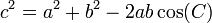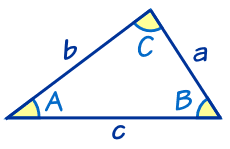a、b 和 c 是三角形的边。 C 边 c 的对角

###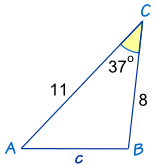余弦定理说： c2 = a2 + b2 − 2ab cos(C) 代入已知值： c2 = 82 + 112 − 2 × 8 × 11 × cos(37º) 计算： c2 = 64 + 121 − 176 × 0.798… 计算： c2 = 44.44... 开平方： c = √44.44 = 6.67 保留两位小数

## 怎样记

 勾股定理： a2 + b2 = c2 （只适用于直角三角形） 余弦定理: a2 + b2 − 2ab cos(C) = c2 （适用于所有三角形）

• 想英语字母 "abc"：a2 + b2 = c2,
• 再来一个 "abc"： 2ab cos(C),
• 放在一起：a2 + b2 − 2ab cos(C) = c2

## 什么时候用

• 已知三角形的两边和两边中间的夹角，求第三边（像上面的例子）
• 已知三角形的三边，求其角度（如以下的例子）

### 例子：角 "C" 是多大？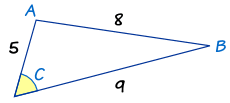开始： c2 = a2 + b2 − 2ab cos(C) 代入 a、b 和 c： 82 = 92 + 52 − 2 × 9 × 5 × cos(C) Calculate: 64 = 81 + 25 − 90 × cos(C) 重排及解: 每边减 25： 39 = 81 − 90 × cos(C) 每边减 81： −42 = −90 × cos(C) 两边对调： −90 × cos(C) = −42 除以 −90： cos(C) = 42/90 取逆余弦： C = cos-1(42/90) 用计算器： C = 62.2° （保留一位小数）

## 其他形式

### 求角度的简易形式

cos(C) = a2 + b2 − c2 2ab

cos(A) = b2 + c2 − a2 2bc

cos(B) = c2 + a2 − b2 2ca

### 例子：用余弦定理（角度形式）来求角 "C"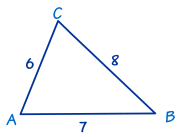• a = 8,
• b = 6 和
• c = 7。

 cos C = (a2 + b2 − c2)/2ab = (82 + 62 − 72)/2×8×6 = (64 + 36 − 49)/96 = 51/96 = 0.53125 C = cos-1(0.53125) = 57.9° 保留一位小数

### a、b 和 c 的形式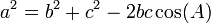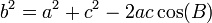### 例子：求长度 "z"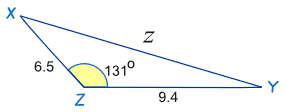开始： c2 = a2 + b2 − 2ab cos(C) x 代替 a、y 代替 b 和 z 代替 c z2 = x2 + y2 − 2xy cos(Z) 代入已知值： z2 = 9.42 + 6.52 − 2×9.4×6.5×cos(131º) 计算： z2 = 88.36 + 42.25 − 122.2 × (−0.656...) z2 = 130.61 + 80.17…… z2 = 210.78…… z = √210.78…… = 14.5 保留一位小数。